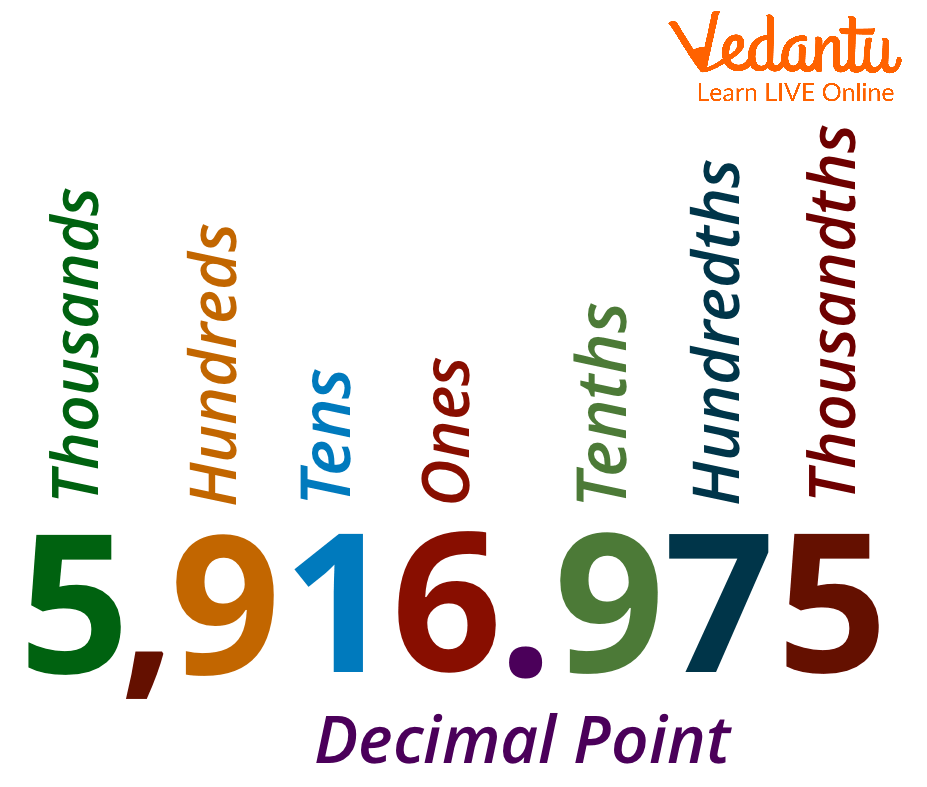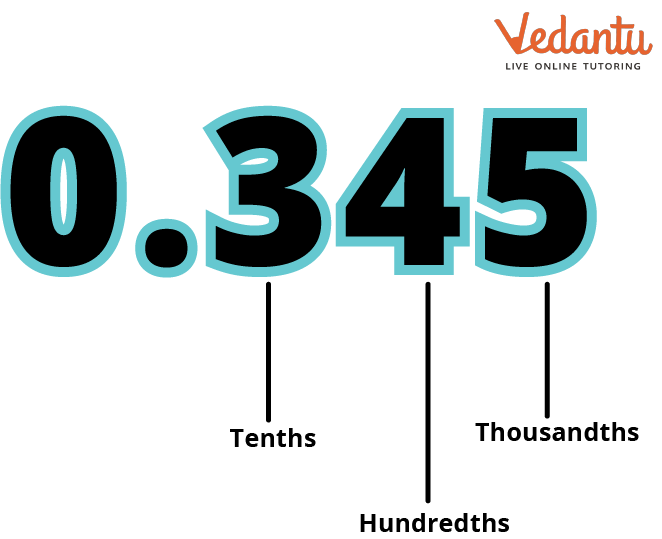Courses
Courses for Kids
Free study material
Free LIVE classes
More

# Thousandth Place in DecimalsLIVE
Join Vedantu’s FREE Mastercalss

## Introduction

Thousand, I hope you kids have heard of this word. You might have heard it from your parents, or teacher or while on your way to school or the park. It might have been used for a thousand rupees note or quantity many more. The fourth position to the left before the decimal point is at the thousands place value.

Whereas today we will learn about the thousandth place. The third position right after the decimal point is at the thousandth place value.

## What is Place Value in Decimal?

A digit has a value called its place value depending where on its position in the number. A decimal point divides a decimal number into a whole number and a fractional part.

From these two statements, we can conclude that the digit's position in a number after the decimal point can be called the place value of a digit in decimal.Place Value in Decimal

## Thousandth Place in Decimal:

The thousandth place in decimals is the third numerical after the decimal.
One-thousandth is one part of the thousand parts or pieces. It does not start with ones. Instead, the number towards the right of the decimal is Tenths, the second digit after the decimal is Hundredths, and the third digit is called Thousandths.

For example, in 6.489 (SIX POINT FOUR EIGHT NINE),

4 (FOUR) is in Tenth place.

8 (EIGHT) is in the Hundredth place.

9 (NINE) is at the Thousandth place.The Thousandth Place of 0.345

## How to Convert the Decimal Fraction 0.825 into a Percentage

To convert a decimal number 0.825 into a percentage, follow the steps:

• The first step is to multiply the decimal by 100.

Let's take the number 0.825

$= \dfrac{825}{1000} \times 100 = 82.5$

The second step is to add the % (PERCENTAGE) sign to the value.

$= 82.5\%$

## Tenth, Hundredth, Thousandth - Chart

 INTEGER PART Thousands 1000 Hundreds 100 Tens 10 Ones 1 DECIMAL PART Tenths $\dfrac{1}{{10}}$ Hundredths $\dfrac{1}{{100}}$ Thousandths $\dfrac{1}{{1000}}$

## 3 Decimal Places Example

The Thousandths place in place value means $\dfrac{1}{{1000}}$ (ONE UPON THOUSAND). The Decimal number is a particular portion of the thousand. Let's take a look at some Thousandths place in decimals examples:

Example 1:

0.033 (ZERO POINT ZERO THREE THREE) is also represented as $\dfrac{{33}}{{1000}}$ (THIRTY-THREE UPON THOUSAND) in fraction. It signifies that it is 33 over one thousand.

Example 2:

0.087 (ZERO POINT ZERO EIGHT SEVEN) is also represented as $\dfrac{{87}}{{1000}}$ (EIGHTY-SEVEN UPON THOUSAND) in fraction. It signifies that it is 87 over one thousand.

Example 3:

0.002 (ZERO POINT ZERO ZERO TWO) is also represented as $\dfrac{2}{{1000}}$ UPON THOUSAND) in fraction. It signifies that it is 2 over one thousand.

## Conclusion

The value that a digit in a number represents depending upon its position is known as place value. The third position to the decimal's right is the thousandth place.

Now, that you guys know what we really mean by a thousandth. Then we can easily understand that if there are 1000 squares equal on a grid and if we spilt one of the squares into 10 equal squares, then one of such small squares will represent the thousandth, which is 1 over one thousand.

Last updated date: 25th Sep 2023
Total views: 92.7k
Views today: 1.92k

## FAQs on Thousandth Place in Decimals

1. What is the significance of place value?

The foundation for regrouping, multiple-digit multiplication, and other operations in the decimal system is set out through place value, which also serves as a starting point for learning other base systems.

2. How may place value be used in daily life?

Place value helps in decision-making for everyday issues including pricing, weight, distances, and timing. Our system of numbers is based on the Base Ten system. Base ten represents the ten base of our number system.

3. What are some place value facts?

Place value is the value that a digit in a number represents based on where it is placed in the number. For example, the place value of 7 in 9,712 is 700, which is 7 hundred. The place value of 7 in 7,689 is, however, 7 thousand, or 7,000.